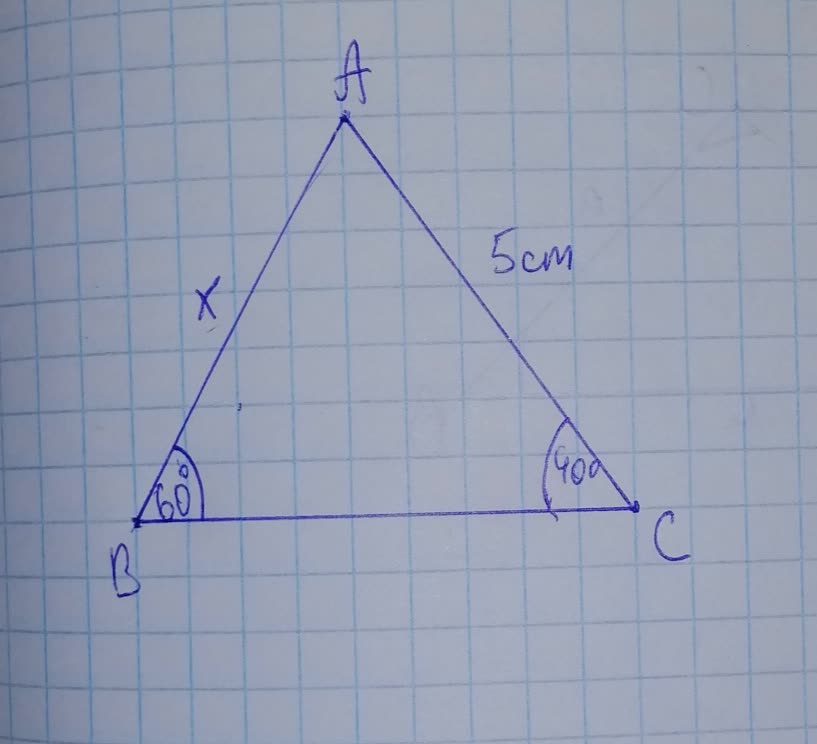# The following diagram shows a triangle ABS, where BC=5,\ \hat{B}=60^{\circ},\kiki195ms 2021-11-20 Answered
The following diagram shows a triangle ABS, where $$\displaystyle{B}{C}={5},\ \hat{{{B}}}={60}^{{\circ}},\ \hat{{{C}}}={40}^{{\circ}}$$. Calculate AB to the nearest whole number.• Questions are typically answered in as fast as 30 minutes

### Plainmath recommends

• Get a detailed answer even on the hardest topics.
• Ask an expert for a step-by-step guidance to learn to do it yourself.Lupe Kirkland
Step 1
The Law of Sines is the relationship between the sides and angles of non-right (oblique) triangles.
Simply, it states that the ratio of the length of a side of a triangle to the sine of the angle opposite that side is the same for all sides and angles in a given triangle.
Step 2
Using hte law of sine in $$\displaystyle\triangle{A}{B}{C}$$
$$\displaystyle\Rightarrow{\frac{{{A}{C}}}{{{\sin{{B}}}}}}={\frac{{{A}{B}}}{{{\sin{{C}}}}}}$$
$$\displaystyle\Rightarrow{\frac{{{5}}}{{{\sin{{60}}}^{{\circ}}}}}={\frac{{{x}}}{{{\sin{{40}}}^{{\circ}}}}}$$
$$\displaystyle\Rightarrow{{\sin{{40}}}^{{\circ}}=}{0.643}$$ and $$\displaystyle{{\sin{{60}}}^{{\circ}}=}{0.866}$$
$$\displaystyle\Rightarrow{x}={\frac{{{5}\times{\sin{{40}}}^{{\circ}}}}{{{\sin{{60}}}^{{\circ}}}}}={\frac{{{5}\times{0.643}}}{{{0.866}}}}={3.71}{c}{m}$$
$$\displaystyle\Rightarrow{x}={4}{c}{m}$$ (to the nearest whole number)